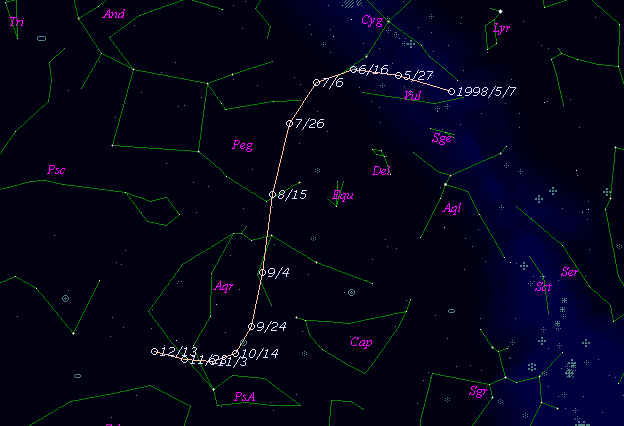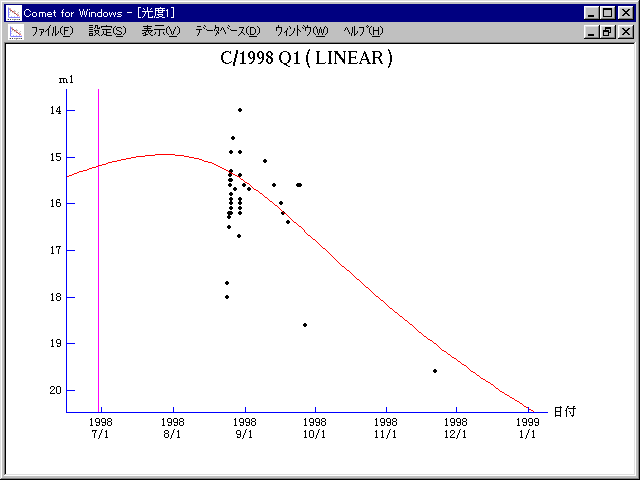# \$B%j%K%"WB@1(B

C/1998 Q1 ( LINEAR )###\$B%W%m%U%#!<%k(B

 \$BH/8+F|(B 1998\$BG/(B8\$B7n(B24\$BF|(B \$BH/8+8wEY(B 17.7\$BEy(B \$BH/8+ Lincoln Laboratory Near-Earth Asteroid Research project

###\$B50F;MWAG(B

```   The following improved orbital elements by Kenji Muraoka, are
from 136 observations 1998 Aug. 24  to  Nov. 22, perturbations
by 9 Planets, Moon and 5 minor planets were taken into account.
The mean residual is +/- 0.58 arc seconds.

Epoch  =  1998 July  6.0  TT       JDT = 2451000.5
T  =  1998 June 29.50742       +/- 0.00056 (m.e.) TT
Peri. =  134.73245                +/- 0.00051
Node  =  159.78466                +/- 0.00037   (2000.0)
Incl. =   32.30563                +/- 0.00033
q  =    1.5778842              +/- 0.0000096 AU
e  =    0.9954500              +/- 0.0000244
1/a  =   +0.0028836              +/- 0.0000154 1/AU
( P  =   6458 years )
orig. 1/a  =   +0.003010   1/AU
fut.  1/a  =   +0.004440   1/AU
```

###\$B@1?^(B###\$B8wEYJQ2=(B

```        m1 = 13.5 + 5 log\$B&\$(B + 10.0 log r
```##### \$B50F;MWAG\$OB<2,7r<#;a\$N7W;;\$K\$h\$k\$b\$N\$G\$9!#(B \$B@1?^\$O(B StellaNavigator Ver.2.0 for Windows (\$B%"%9%H%m%"!<%D(B \$BJTCx(B / \$B%"%9%-!<=PHG6I4)(B) \$B\$G:n@.\$7\$?\$b\$N\$G\$9!#(B \$B8wEY%0%i%U\$O(BComet for Windows\$B\$G:n@.\$7\$?\$b\$N\$G\$9!#(B